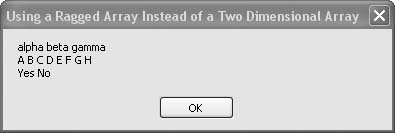# Recipe 8.11. Using a Multivalue Array Instead of a Two-Dimensional Array

#### Problem

You want to store data in a two-dimensional array, but the number of items in each row varies. You don't want to dimension the array for the longest row and waste a lot of space in the array.

#### Solution

Sample code folder: Chapter 08\MultivalueArray

Instead of creating a two-dimensional array, create an array of arrays, sometimes referred to as a multivalue array.

#### Discussion

A two-dimensional array is identified by its single pair of parentheses containing one comma separating the two indexes. A multivalue array has two sets of parentheses, and the contents are stored as one-dimensional arrays stored in the elements of another one-dimensional array. The following code demonstrates a multivalue array containing three string arrays of varying lengths:

` Dim result As New System.Text.StringBuilder Dim multiValue(2)( ) As String Dim counter1 As Integer Dim counter2 As Integer ' ----- Build the multivalue array. multiValue(0) = New String( ) {"alpha", "beta", "gamma"} multiValue(1) = New String( ) _    {"A", "B", "C", "D", "E", "F", "G", "H"} multiValue(2) = New String( ) {"Yes", "No"} ' ----- Format the array for display. For counter1 = 0 To multiValue.Length - 1    For counter2 = 0 To multiValue(counter1).Length - 1       result.Append(multiValue(counter1)(counter2))       result.Append(Space(1))    Next counter2    result.AppendLine( ) Next counter1 MsgBox(result.ToString( )) `

Inside the nested For loops is a line where each string from the array of arrays is accessed to form the results displayed in Figure 8-11. Two pairs of parentheses are used to index the specific string stored in the multivalue array:

` multiValue(counter1)(counter2) `

##### Figure 8-11. Using multivalue arrays to store a variable number of items in each row of a two-dimensional arrayA true two-dimensional array element would be accessed with a pair of indexes within one set of parentheses, as in the following:

` twoDimArray(counter1, counter2) `Visual Basic 2005 Cookbook: Solutions for VB 2005 Programmers (Cookbooks (OReilly))
ISBN: 0596101775
EAN: 2147483647
Year: 2006
Pages: 400

Similar book on Amazon